Courses

# RD Sharma Solutions -Ex-21.1, Surface Area And Volume Of Sphere, Class 9, Maths Class 9 Notes | EduRev

## Class 9 : RD Sharma Solutions -Ex-21.1, Surface Area And Volume Of Sphere, Class 9, Maths Class 9 Notes | EduRev

The document RD Sharma Solutions -Ex-21.1, Surface Area And Volume Of Sphere, Class 9, Maths Class 9 Notes | EduRev is a part of the Class 9 Course RD Sharma Solutions for Class 9 Mathematics.
All you need of Class 9 at this link: Class 9

Q1. Find the surface area of a sphere of radius:

(i) 10.5cm (ii) 5.6cm (iii) 14cm

Sol.

(i) Given Radius = 10.5 cm

Surface area =  4πr2

= 4×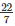×(10.5)2

= 1386cm2

Surface area =  4πr2

= 4××(5.6)2

= 394.24cm2

Surface area =  4πr2

= 4××(14)2

= 2464cm2

Q2. Find the surface area of a sphere of diameter:

(i) 14cm (ii) 21cm (iii) 3.5cm

Sol.

(i) Given Diameter = 14 cm

Radius =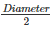=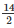= 7cm

Surface area =  4πr2

= 4××(7)2

= 616cm2

(ii) Given Diameter = 21cm

Radius ==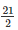= 10.5cm

Surface area =  4πr2

= 4××(10.5)2

= 1386cm2

(iii) Given diameter = 3.5cm

Radius ==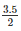= 1.75cm

Surface area =  4πr2

= 4××(1.75)2

= 38.5cm2

Q3. Find the total surface area of a hemisphere and a solid hemisphere each of radius 10cm (∏ = 3.14)

Sol.

The surface area of the hemisphere =  2πr2

=  2×3.14×(10)2

= 628cm2

The surface area of solid hemisphere =   2πr2

=  3×3.14×(10)2

= 942cm2

Q4. The surface area of a sphere is 5544cm2, find its diameter.

Sol.

Surface area of a sphere is 5544cm2

4πr2 = 5544

4×3.14×(r)2 = 5544

r2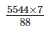r =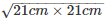r = 21cm

= 2(21)  = 42cm

Q5. A hemispherical bowl made of brass has inner diameter 10.5cm. Find the cost of tin plating it on the inside at the rate of Rs.4 per 100cm2.

Sol.

Inner diameter of hemispherical bowl = 10.5cm

Radius == 5.25cm

Surface area of hemispherical bowl =  2πr2

= 2×3.14×(5.25)2

= 173.25cm2

Cost of tin plating 100cm2 area = Rs.4

Cost of tin plating 173.25cm2 area = Rs.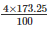= Rs. 6.93

Thus, the cost of tin plating the inner side of hemispherical bowl is Rs.6.93

Q6. The dome of a building is in the form of a hemisphere. Its radius is 63dm. Find the cost of painting it at the rate of Rs.2 per sq m.

Sol.

Dome radius = 63dm = 6.3m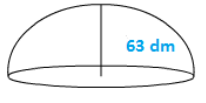Inner surface area of dome =  2πr2

= 2×3.14×(6.3)2

= 249.48m2

Now, cost of 1m2 = Rs.2

Therefore cost of 249.48m2 = Rs. (249.48×2)  = Rs.498.96

Q7. Assuming the earth to be a sphere of radius 6370km, how many square kilometers is the area of the land if three-fourths of the earth’s surface is covered by water?

Sol.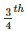of earth surface is covered by water

Therefore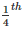of earth surface is covered by land

Therefore Surface area covered by land =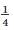×4πr2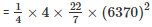= 127527400km2

Q8. A cylinder of same height and radius is placed on top of a hemisphere. Find the curved surface area of the shape if the length of the shape is 7cm.

Sol.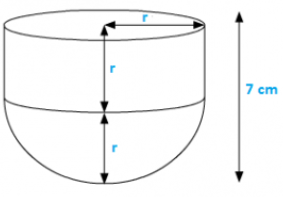Given length of the shape = 7cm

But length = r+r

2r = 7cm

r = 3.5cm

Also; h = r

Total surface area of shape =  2πrh+2πr2

= 2πrr+2πr2

=  2πr2+2πr2

= 4××(3.5)2  = 154cm2

Q9. A wooden toy is in the form of a cone surmounted on a hemisphere. The diameter of the base of the cone is 16cm and its height is 15cm. Find the cost of painting the toy at Rs.7 per 100cm2

Sol.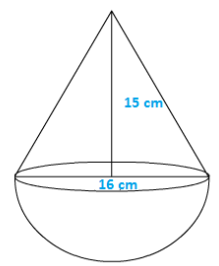Diameter of cone = 16cm

Height of cone = 15cm

Slant height of cone =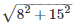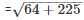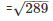= 17cm

Therefore Total curved surface area of toy

=  πrl+2πr2

=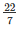×8×17+2××82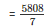cm2

Now, cost of 100cm2 = Rs.7

1cm2 = Rs.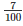Hence cost of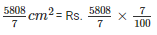= Rs. 58.08

Q10. a storage tank consists of a circular cylinder with a hemisphere adjoined on either end. If the external diameter of the cylinder be 1.4m and its length is 8m, find the cost of painting it on the outside at the rate of Rs.10 per m2.

Sol.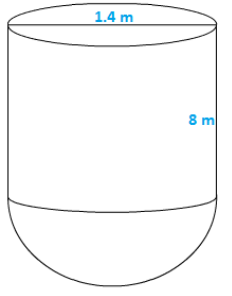Diameter of a cylinder = 1.4m

Therefore radius of cylinder =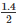= 0.7m

Height of cylinder = 8m

Therefore surface area of tank =  2πrh+2πr2

= 2××0.7×8+2××(0.7)2

=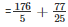= 38.28m2

Now cost of 1m2 = Rs.10

Therefore cost of 38.28m2 = Rs. 382.80

Q11. The diameter of the moon is approximately one-fourth of the diameter of the earth. Find the ratio of their surface areas.

Sol.

Let the diameter of the earth be d

Then,

Diameter of moon will be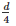Radius of earth =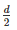Radius of moon =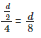Surface area of moon =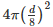Surface area of earth =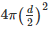Required ratio =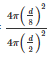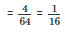Thus the required ratio of the surface areas is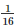Q12. A hemispherical dome of a building needs to be painted. If the circumference of the base of the dome is 17.6cm, find the cost of painting it, given the cost of painting is Rs.5 per 100cm2

Sol.

Given that only the rounded surface of the dome to be painted, we would need to find the curved surface area of the hemisphere to know the extent of painting that needs to be done.

Now, circumference of the dome = 17.6cm

Therefore 2πr = 17.6×r = 17.6m

So, the radius of the dome =  2πr2

=  2××2.8×2.8

= 49.28m2

Cost of painting 100cm2 is Rs.5

So, the cost of painting 1m2 = Rs.500

Therefore the cost of painting the whole dome = Rs. 500×49.28

= Rs. 24640

Q13. The front compound wall of a house is decorated by wooden spheres of diameter 21 cm, placed on small supports as shown in the figure. Eight such spheres are used for this purpose and are to be painted silver. Each support is a cylinder of radius 1.5cm and height 7cm and is to be painted black. Find the cost of paint required if silver paint costs 25 paise per cm2.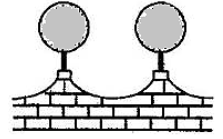Sol.

Wooden sphere radius =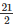= 10.5cm

Surface area of a wooden sphere =  4πr2 = 4××(10.5)2 = 1386cm2

Radius r of cylindrical support = 1.5cm

Height h of cylindrical support = 7cm

Curved surface area of cylindrical support =  2πrh = 2××1.5×7 = 66cm2

Area of circular end of cylindrical support =  πr2 =×(1.5)2 = 7.07cm2

Area to be painted silver =  8(1386−7.07)cm2 = 8(1378.93)cm2 = 11031.44cm2

Cost occurred in painting silver colour =  (11034.44×0.25) = Rs.2757.86

Area to be painted black =  (8×66)cm2 = 528cm2

Cost occurred in painting black colour = (528×5.05) = Rs.26.40

Therefore total cost in painting = Rs.2757.86 + Rs.26.40 = Rs.2784.26

Offer running on EduRev: Apply code STAYHOME200 to get INR 200 off on our premium plan EduRev Infinity!

91 docs

,

,

,

,

,

,

,

,

,

,

,

,

,

,

,

,

,

,

,

,

,

,

,

,

,

,

,

,

,

,

;